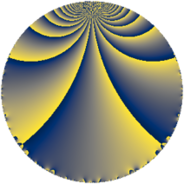Properties

 Label 64.3.fLevel $64$ Weight $3$ Character orbit 64.f Rep. character $\chi_{64}(15,\cdot)$ Character field $\Q(\zeta_{4})$ Dimension $6$ Newform subspaces $1$ Sturm bound $24$ Trace bound $0$

Related objects

Defining parameters

 Level: $$N$$ $$=$$ $$64 = 2^{6}$$ Weight: $$k$$ $$=$$ $$3$$ Character orbit: $$[\chi]$$ $$=$$ 64.f (of order $$4$$ and degree $$2$$) Character conductor: $$\operatorname{cond}(\chi)$$ $$=$$ $$16$$ Character field: $$\Q(i)$$ Newform subspaces: $$1$$ Sturm bound: $$24$$ Trace bound: $$0$$

Dimensions

The following table gives the dimensions of various subspaces of $$M_{3}(64, [\chi])$$.

Total New Old
Modular forms 40 10 30
Cusp forms 24 6 18
Eisenstein series 16 4 12

Trace form

 $$6 q + 2 q^{3} - 2 q^{5} + 4 q^{7} + O(q^{10})$$ $$6 q + 2 q^{3} - 2 q^{5} + 4 q^{7} + 18 q^{11} - 2 q^{13} - 4 q^{17} - 30 q^{19} - 20 q^{21} - 60 q^{23} - 64 q^{27} - 18 q^{29} - 4 q^{33} + 100 q^{35} + 46 q^{37} + 196 q^{39} + 114 q^{43} + 66 q^{45} - 46 q^{49} - 156 q^{51} + 78 q^{53} - 252 q^{55} - 206 q^{59} + 30 q^{61} + 12 q^{65} + 226 q^{67} - 116 q^{69} + 260 q^{71} + 238 q^{75} - 212 q^{77} + 86 q^{81} - 318 q^{83} - 212 q^{85} - 444 q^{87} - 188 q^{91} - 32 q^{93} - 4 q^{97} + 226 q^{99} + O(q^{100})$$

Decomposition of $$S_{3}^{\mathrm{new}}(64, [\chi])$$ into newform subspaces

Label Dim. $$A$$ Field CM Traces $q$-expansion
$a_{2}$ $a_{3}$ $a_{5}$ $a_{7}$
64.3.f.a $6$ $1.744$ 6.0.399424.1 None $$0$$ $$2$$ $$-2$$ $$4$$ $$q-\beta _{5}q^{3}+(-1-\beta _{1}-\beta _{3}-\beta _{5})q^{5}+\cdots$$

Decomposition of $$S_{3}^{\mathrm{old}}(64, [\chi])$$ into lower level spaces

$$S_{3}^{\mathrm{old}}(64, [\chi]) \cong$$ $$S_{3}^{\mathrm{new}}(16, [\chi])$$$$^{\oplus 3}$$